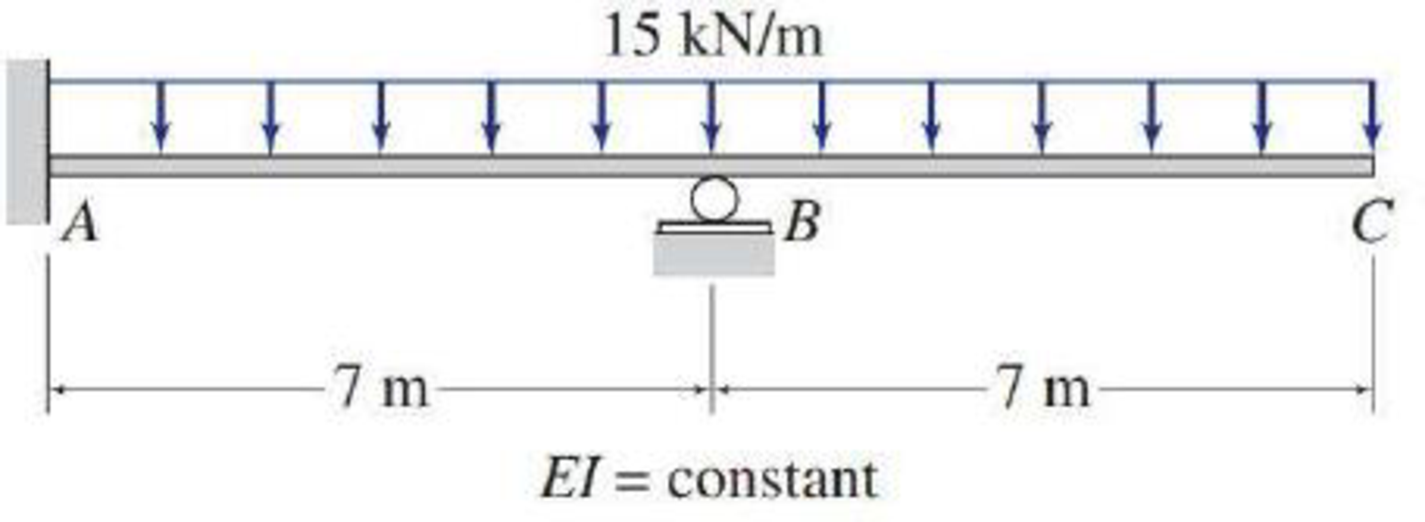# Solve Problem 13.15 by the method of least work. See Fig. P13.15. 13.13 through 13.25 Determine the reactions and draw the shear and bending moment diagrams for the structures shown in Figs. P13.13–P13.25 using the method of consistent deformations. FIG. P13.15, P13.58

#### Solutions

Chapter
Section
Chapter 13, Problem 58P
Textbook Problem
3 views

## Solve Problem 13.15 by the method of least work. See Fig. P13.15.13.13 through 13.25 Determine the reactions and draw the shear and bending moment diagrams for the structures shown in Figs. P13.13–P13.25 using the method of consistent deformations.FIG. P13.15, P13.58To determine

Find the reactions and sketch the shear and bending moment diagrams for the given beam using method of least work.

### Explanation of Solution

Given information:

Apply the sign conventions for calculating reactions, forces and moments using the three equations of equilibrium as shown below.

• For summation of forces along x-direction is equal to zero (Fx=0), consider the forces acting towards right side as positive (+) and the forces acting towards left side as negative ().
• For summation of forces along y-direction is equal to zero (Fy=0), consider the upward force as positive (+) and the downward force as negative ().
• For summation of moment about a point is equal to zero (Matapoint=0), consider the clockwise moment as negative and the counter clockwise moment as positive.

Calculation:

Show the free body diagram of the beam as shown in the Figure 1.

Refer Figure 1.

Consider the horizontal and vertical reaction at A are denoted by Ax and Ay.

Consider the moment at A is denoted by MA.

Consider the vertical reaction at B is denoted by By.

The reactions acting in the beam is 4.

The number of Equilibrium reaction is 3.

The degree of indeterminacy of the beam is 1.

Take the vertical reaction at B as the redundant.

Consider a section at a distance x from support C as shown in Figure 2.

Refer Figure 2.

Determine the bending moment at x for segment CB.

Mx=15×x×x2=7.5x2

Differentiate with respect to By.

MxBy=0

Determine the bending moment at x for segment BA.

Mx=15×x×x2+By(x7)=7.5x2+By(x7)

Differentiate with respect to By.

MxBy=x7

Calculate the reaction at B (By) using the method of least work as shown below.

UBy=(MxBy)MxEIdx=0        (1)

Substitute 7.5x2 for Mx and 0 for MxBy for the span CB and 7.5x2+By(x7) for Mx and x7 for MxBy for the span BA.

07(0)7.5x2EIdx+714(x7)(7.5x2+By(x7))EIdx=01EI714(7.5x2(x7)+By(x7)2)dx=01EI714(7.5x3+52.5x2+By(x2+4914x))dx=01EI714(7.5x3+52.5x2)dx+ByEI714(x2+4914x)dx=0

(7

### Still sussing out bartleby?

Check out a sample textbook solution.

See a sample solution

#### The Solution to Your Study Problems

Bartleby provides explanations to thousands of textbook problems written by our experts, many with advanced degrees!

Get Started

Find more solutions based on key concepts
What are the units of mass and weight in U.S.?

Engineering Fundamentals: An Introduction to Engineering (MindTap Course List)

Should Gladys call the legal authorities? Which agency should she call?

Principles of Information Security (MindTap Course List)

No single person or government agency controls or owns the Internet.

Enhanced Discovering Computers 2017 (Shelly Cashman Series) (MindTap Course List)

Convert P = 5.00 atm into Pa, bar, and psia.

Fundamentals of Chemical Engineering Thermodynamics (MindTap Course List)

Saw guides should be mounted ________ above the part on a vertical saw.

Precision Machining Technology (MindTap Course List)

In what way are policies different from standards?

Management Of Information Security

What two types of modulation does DSL use to carry data?

Network+ Guide to Networks (MindTap Course List)

If your motherboard supports ECC DDR3 memory, can you substitute non-ECC DDR3 memory?

A+ Guide to Hardware (Standalone Book) (MindTap Course List)